# Determining and Using Newton's Second Law

3 teachers like this lesson
Print Lesson

## Objective

Students will be able to state Newton’s 2nd Law and quantitatively describe the relationship between acceleration, net force and mass.

#### Big Idea

After analyzing lab data, students determine the equation for Newton's second law.

## Mega-Whiteboard Modified Atwood Lab Discussion

25 minutes

The goal of this lesson is for students to quantitatively understand the relationship between net force & acceleration and mass & acceleration. Students collected data from the Modified Atwood Lab in the previous lesson and analyzed the force & acceleration data and mass & acceleration data graphically to determine mathematical models for each relationship (SP5). In this lesson, students share out the results they collected from their lab and analysis in a discussion. The analysis is where students make claims about the relationships and supporting them with the evidence from the lab (SP6) to develop a model for Newton's second law (SP2). Students then apply Newton's second law in a series of online simulations.

To start out class, I ask for a brief recap of what the students learned about the relationships between the net force & acceleration and the net force & mass by having them put all of their mathematical models on the board. I also ask students to finish up their lab summaries and to put up their results on the whiteboard so we can analyze them as a class.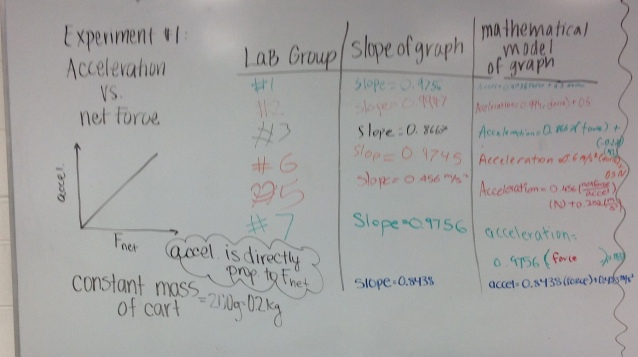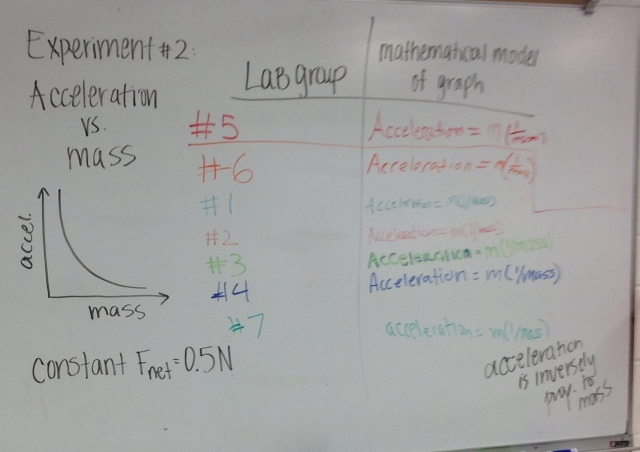Students see from their own lab data analysis compared to everyone's data that net force and acceleration are directly proportional and that mass and net force are inversely proportional. I show students that when we put these two relationships together it makes an equation showing Newton's second law. Below are videos that show the class discussion in how we look at each set of data and use that to come up with the equation for Newton's second law.

In this first video, we develop the relationship between acceleration and net force.

In this video, we develop the relationship between acceleration and mass.

In this video, we put the relationships together to make a mathematical model for Newton's second law.

## Second Law Simulations

40 minutes

After the students have developed the two relationships from Newton's second law, I have them work with partners to complete four simulations that give them more experience with these relationships. I have them complete the Simulation Worksheet that has them answer questions with each online simulation to allow them to see Newton's second law relationships in multiple situations. I like this activity because it is very visual and helps a lot of students see a better picture of the relationships.

I have students work in pairs and have them alternate who is working on the Chromebook. I like for them to use a shared resource of one Chromebook so that one person works on the simulation while the other person asks the questions and both students answer the questions together.

The four simulations links are shown below:

Alien Space Ship Simulation- This simulation allows students to add passengers to change the mass of each space ship to see what happens to the acceleration. With the three space ships, students can compare the accelerations of different ratios of force to mass.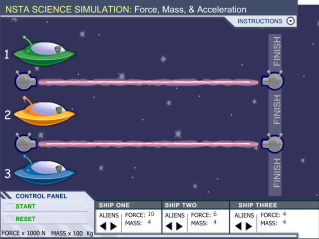Grocery Cart Simulation- This simulation is great because students are able to select a force by selecting a person and a mass by selecting a grocery cart. The numbers and units are put into the equation that is shown in the bottom left to show how the units cancel and the numbers divide. After showing the calculations, the shopper actually accelerates across the screen.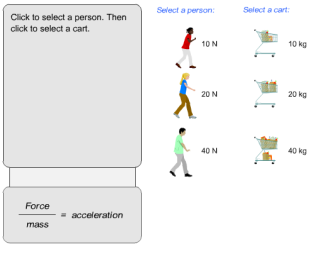Horizontal Balloon Simulation- This simulation helps students to look at a different example of Newton's 2nd Law using the balloons to provide the force and adding mass with the washers.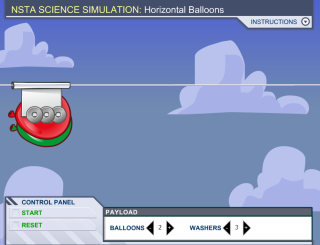Vertical Balloon Simulation- This simulation is good for students to see the contrast to the horizontal balloon simulation. In this simulation, I ask students to see what the minimum amount of balloons would need to be to lift it off the ground so they have to think about have a greater acceleration than acceleration due to gravity since it is vertical.These simulations really help students to have visual representations of Newton's second law right after we have developed the relationships between force & acceleration and mass & acceleration.

## Stoplight Exit Slip

5 minutes

After the simulations, each students takes a post-it note from the resource bin at the center of the table and gets to chose from the red, yellow or green light. They must complete the sentence starter on the light they choose.

The Red Light: Today my learning stopped because...

The Yellow Light: Today I considered a question, new idea or perspective...

The Green Light Today I learned __________ because...

When they complete their sentence, they stick the post-it note on whatever light they choose. The stoplight is at the front of the room whenever they finish writing their response to a light.

I do this to get an idea of where students are at in learning the material in a way that they can anonymously express exactly what they are learning or exactly what they are struggling with.